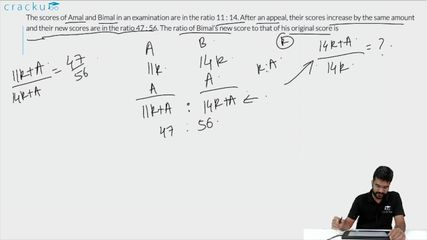Question 71

# The scores of Amal and Bimal in an examination are in the ratio 11 : 14. After an appeal, their scores increase by the same amount and their new scores are in the ratio 47 : 56. The ratio of Bimal’s new score to that of his original score is

Solution

Let the score of Amal and Bimal be 11k and 14k
Let the scores be increased by x
So, after increment, Amal's score =  11k + x and Bimal's score = 14k + x
According to the question,
$$\dfrac{\text{11k + x}}{\text{14k + x}}$$ = $$\dfrac{47}{56}$$
On solving, we get x = $$\dfrac{42}{9}$$k
Ratio of Bimal's new score to his original score

= $$\dfrac{\text{14k + x}}{\text{14k}}$$

=$$\dfrac{14k +\frac{42k}{9}}{14k}$$

=$$\dfrac{\text{168k}}{\text{14*9k}}$$

=$$\dfrac{4}{3}$$

Hence, option A is the correct answer.

### View Video Solution